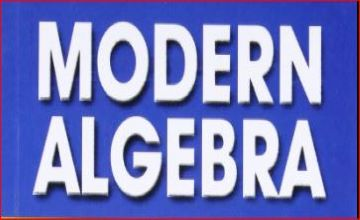Modern (Abstract) Algebra for UPSC

Ramanasri IAS Maths Optional is teaching Modern Algebra for UPSC. Ramanasri Sir is teaching all the 13 Modules. For example from 1st Module Linear Algebra to Last i.e.,13th Module Mechanics & Fluid Dynamics. This Modern Algebra Module is Difficulty Level like Mechanics and Fluid Dynamics, Statics and Dynamics, Modern Algebra, Real Analysis. In Ramanasri IAS Ramanasri Sir is delivering Best Lectures for these all four Modules in addition to the rest of 9 Modules. The rest of the 9 Modules 5 Modules are very easy like Vector Analysis, Complex Analysis, Linear Programming, Numerical Analysis and Computer Programming, Calculus.
Medium Level Modules are Ordinary Differential Equations, Partial Differential Equations, Analytical Geometry.

In this Below we are giving Modern Algebra for UPSC Maths Syllabus. And also Modern Algebra for IFS or IFoS Maths Syllabus.

Modern Algebra for UPSC Maths Syllabus | IAS Maths Syllabus

Groups, subgroups, cyclic groups, cosets, Lagrange’s Theorem, normal subgroups, quotient groups, homomorphism of groups, basic isomorphism theorems, permutation groups, Cayley’s theorem. Rings, subrings and ideals, homeomorphisms of rings; Integral domains, principal ideal domains, Euclidean domains, and unique factorization domains; Fields, quotient fields.

Modern Algebra for IFS Maths Syllabus | IFoS Maths Syllabus.

Groups, subgroups, normal subgroups, homomorphism of groups, quotient groups basic isomorphism theorems, Sylow’s group, permutation groups, Cayley theorem. Rings and ideals, principal ideal domains, unique factorization domains, and Euclidean domains. Field extensions, finite fields.

Modern Algebra Common topics for UPSC Maths Syllabus | IAS Maths Syllabus & IFS or IFoS Maths Syllabus

Groups, subgroups, cyclic groups, cosets, Lagrange’s Theorem, normal subgroups, quotient groups, homomorphism of groups, basic isomorphism theorems, permutation groups, Cayley’s theorem. Rings, subrings and ideals, homeomorphisms of rings; Integral domains, principal ideal domains, Euclidean domains, and unique factorization domains; Fields, quotient fields.

List of topics of Modern Algebra for ONLY UPSC Maths Syllabus | IAS Maths Syllabus

cosets, Lagrange’s Theorem, cyclic groups, homeomorphisms of rings; Integral domains, Fields, quotient fields.

List of topics of Modern Algebra for ONLY IFS Maths Syllabus | IFoS Maths Syllabus

Sylow’s group, sub rings, Field extensions, finite fields..

Click on this below links to know more details.

Click on this below links to know more details about RAMANASRI IAS You Tube Channel.